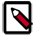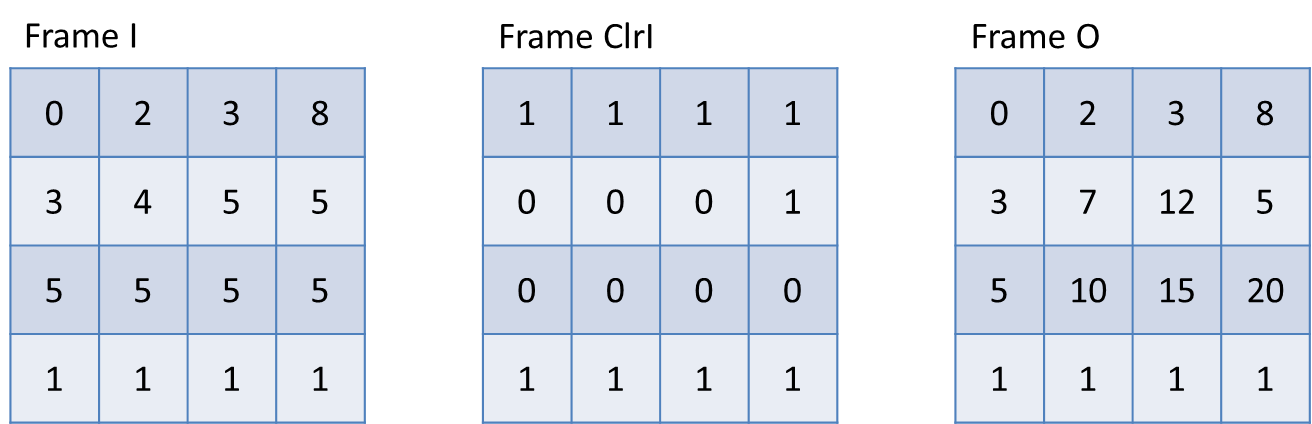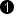## Operator RowSum

This operator calculates the sum of the pixel values for each image row. Each pixel value at the output represents the current sum of the image row. Thus, each pixel is replaced by the sum determined so far.

 Resource ConsumptionThe operator's FGPA resource consumption strongly increases with the parallelism. We recommend to use low parallelism at this operator. In many use cases of this operator, the parallelism can be reduced by adding up the parallel components prior this operator using SplitParallel and ADD.

With every new input row the sum for summations starts from zero. Additionally, it is possible to clear the current row sum manually when the ClrI link is set to 1. When a manual clearing is not required, you must ensure a constant 0 at ClrI.

The following example shows the relation of input values to output values.Often, this operator is used in conjunction with operator RemovePixel to use only the last column containing the results of the full frame. Note that the operator will require additional resources with increased parallelism. If only the sum of each row without intermediate results is required, it is possible to determine the sum of the parallel words first.

Image Restrictions

• Image Size

Varying line lengths and empty lines are allowed in non-empty images.

• Empty Images

Empty images, i.e. images with no pixels, are allowed. The operator will output an empty image.

### I/O Properties

Property Value
Operator Type O
ClrI, Clear Input

Bit Width [1, 64] unsigned, [2, 64] signed 1 autoArithmetic {unsigned, signed} unsigned as I
Parallelism any as I as I
Kernel Columns 1 as I as I
Kernel Rows 1 as I as I
Img Protocol {VALT_IMAGE2D, VALT_LINE1D} as I as I
Color Format VAF_GRAY as I as I
Color Flavor FL_NONE as I as I
Max. Img Width any as I as I
Max. Img Height any as I as I
 The output bit width is automatically determined from the input bit width and maximum image width. The output bit width is determined by The output bit width must not exceed 64 Bit.

None

### Examples of Use

The use of operator RowSum is shown in the following examples: